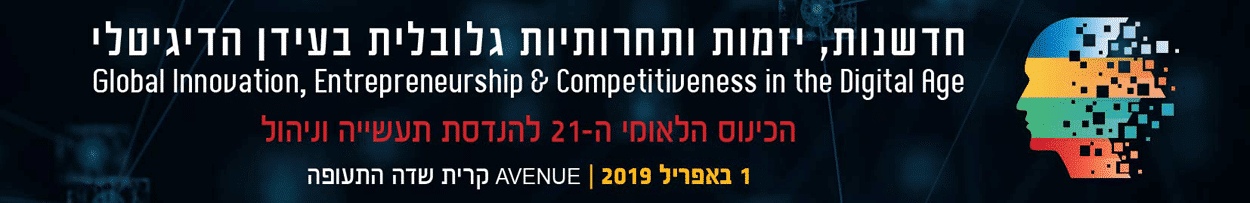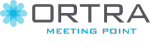# OPTIMIZING PERFORMANCE IN DIVERGE INTEREST TACIT COORDINATION GAMES USING AN AUTONOMOUS AGENT.

Department of Industrial Engineering and Management, Ariel University, ישראל

The Social Value Orientation (SVO) theory describes the preferences or motivations of decision makers when allocating joint resources between themselves and another person. Hence, the SVO has a significant impact on the decision-making process. This study explores the effect of the SVO on playing strategies in diverge interest tacit coordination games. Tacit coordination games are games in which communication between the players is not possible. When the reward in this type of games is not symmetric and the payoff is not equal those games are considered to be diverge interest tacit coordination games. Game theory can only partially explain choice behavior in tacit coordination games because of the existence of multiple Nash equilibria. However, it was shown that people managed to converge on "focal points", which more prominent solutions with salient features which attract the player`s attention. In the first part of the study, we have created a statistical model that can predict the probability of a particular player to converge on a focal point solution based on the SVO and the specific game features. The players were presented with the bargaining table, a diverge interest tacit coordination game. In the second part of the study, we constructed an autonomous agent on the basis of the statistical model developed in the first part. The aim of the agent was to achieve an optimal solution by maximizing the average number of points gained across all games with a minimal variance as possible. The decision of the agent was stochastic. That is, it was based on assessing the probability of a player converging on a focal point. Next, the agent selected stochastically between one of two possible solutions: a greedy solution or a focal point solution. The distribution of the number of points obtained by the autonomous agent incorporating the SVO in the model was better than the results obtained by the team players who played against each other (i.e. the distribution associated with the agent had a higher mean value and a smaller variance). Moreover, the distribution of points gained by the agent was better than any of the separate strategies the agent could choose from, namely, always choosing a greedy solution, or the focal point solution. To the best of our knowledge, this is the first attempt to construct an intelligent agent that maximizes its utility by modeling the probability of implementing a focal point solution.

החברה המארגנת: ארטרא בע"מ, רחוב יגאל אלון 94 תל אביב 6109202 טלפון: 03-6384444, פקס: 6384455–03
iem@ortra.com מייל לשאלות